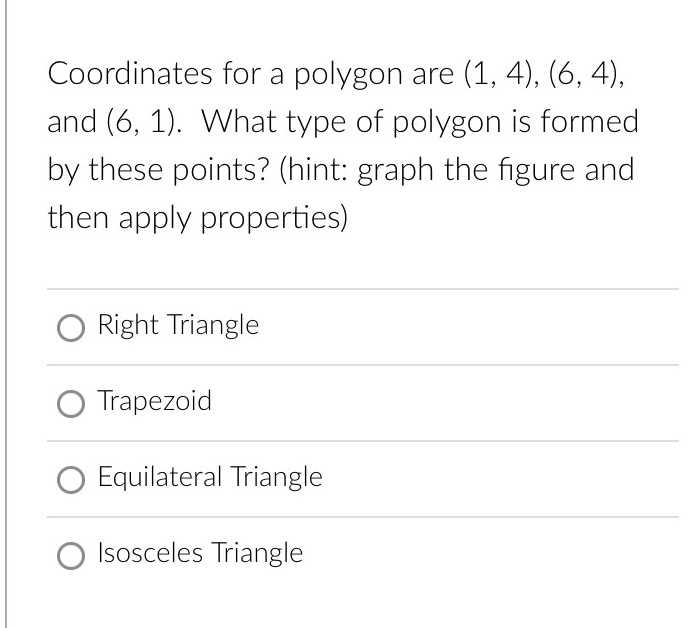### Still have math questions?Coordinates for a polygon are $$( 1,4 ) , ( 6,4 )$$ , and $$( 6,1 )$$ . What type of polygon is formed by these points? (hint: graph the figure and then apply properties) Right Triangle Trapezoid Equilateral Triangle Isosceles Triangle